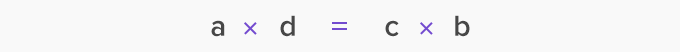Cross Multiplication - Definition with Examples

The Complete K-5 Math Learning Program Built for Your Child

• 30 Million Kids

Loved by kids and parent worldwide

• 50,000 Schools

Trusted by teachers across schools

• Comprehensive Curriculum

Aligned to Common Core

Cross Multiplication

In cross-multiplication, we multiply the numerator of the first fraction with the denominator of the second fraction and the numerator of the second fraction with the denominator of the first fraction.

Example:Comparing fractions using cross-multiplication

Unlike fractions can be compared using cross-multiplication.

Example:

Compare 37 and 5using cross-multiplication.

To compare two fractions with different denominators, we make their denominators the same.

We do it by changing the denominators to the product of both the denominators.So, the denominator of both the fractions becomes 7 × 8 = 56

Now, we use cross-multiplication, to find the numerators. First, we multiply the numerator of the first fraction with the denominator of the second fraction.3 × 8 = 24

So, the first fraction becomes: 2456

Next, we multiply the numerator of the second fraction by the denominator of the first fraction.5 × 7 = 35

So, the second fraction becomes: 3556

Since  2456 3556

Therefore, 37 < 58

Example: Which is bigger, 25 or 34 ?

Using cross-multiplication we find,2 × 4 = 8 and 3 × 5 = 15

As, 8 < 15

Therefore, 25 < 34

Solving equations involving ratios using cross-multiplication

We can use cross-multiplication to solve an equation with 2 ratios and find the value of the variable.

If we have acd , then

where b and d are not equal to zero, then we cross multiply asto get:We can use cross-multiplication to find the value of a variable in an equation involving ratios, as shown in the example.

Example: If 8 candle holders cost \$40. How much will 12 such candle stands cost?

Cost of 8 candle holders = \$40

Cost of 1 candle holder = 408................................................(i)

Let the cost of 12 candle holders be x

Therefore, the cost of 1 candle holder will be  x12....................(ii)

Equating (i) and (ii) we get

408 = x12

On cross-multiplying:

40 × 12 = 8 × x

4808 = x

60 = x

Therefore, the cost of 12 candle holders is \$60

 Fun Facts Cross-multiplication can be used to add or subtract unlike fractions quickly. Cross-multiplication is also referred to as butterfly method.

Won Numerous Awards & Honors